Get instant live expert help with Excel or Google Sheets“My Excelchat expert helped me in less than 20 minutes, saving me what would have been 5 hours of work!”

#### Post your problem and you’ll get Expert help in seconds.

Your message must be at least 40 characters
Our professional Expert are available now. Your privacy is guaranteed.

# Highlight duplicate values

Excel has some very effective ways to deal with duplicate values. Highlighting duplicates is one such feature. We can easily highlight duplicate values using conditional formatting and the COUNTIF function. In this tutorial, we will learn how to highlight duplicate values in Excel.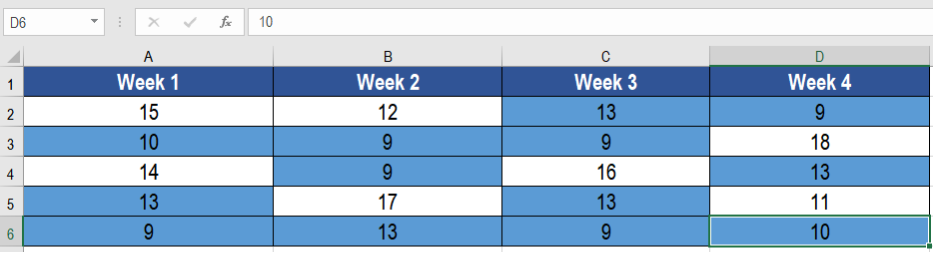Figure 1. Example of How to Highlight Duplicate Values

## Generic Formula

`=COUNTIF(Range,A1)>1`

## How the Formula Works

Here, we are using conditional formatting to highlight the duplicate values. The function in use is COUNTIF. The COUNTIF function counts the occurrence of each value in the range. If a value exists more than once, COUNTIF returns TRUE and invokes the formatting rule. .

We use conditional formatting to highlight the cells. Conditional formatting has some preset rules. Still, we are using a custom formula for more flexibility. Excel evaluates the formula used in conditional formatting. It evaluates the formula relative to the cell that is active. This evaluation is done in the selection of the time the rule was created. We are using COUNTIF in the range with an absolute address. But the first cell is relative. Therefore, the range remains unchanged. But, the relative cell A1 changes for each iteration.

## Setting Up Data

The following example contains a temperature database during the weekdays of December. Columns A, B, C and D has the name of the winners for each week.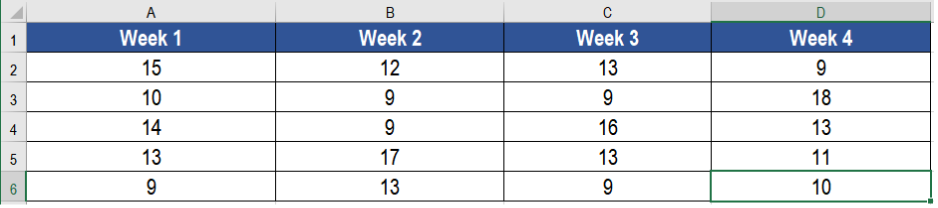Figure 2. The Data Set

To highlight duplicate values, we need to:

• Select cells A2 to D6 with your mouse.
• From the home tab in the ribbon, go to Conditional Formatting > New Rule.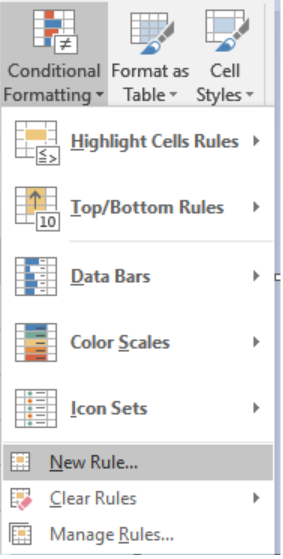Figure 3. Example of how to Apply Conditional Formatting

• Click on Use a formula to determine which cells to format.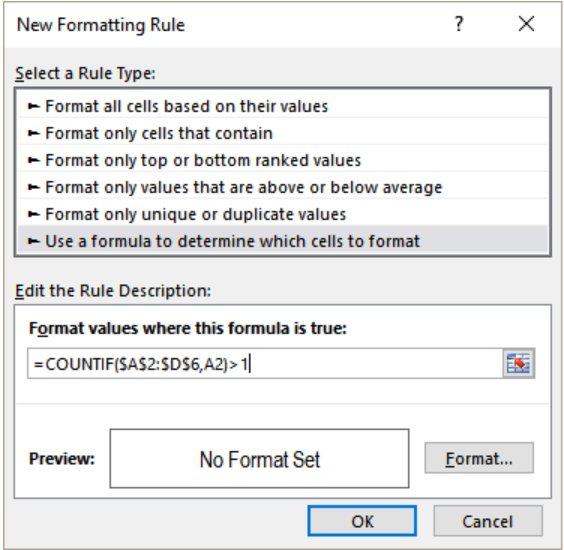Figure 4. Applying the Formula

• In the Format values where this formula is true box, we need to assign the formula `=COUNTIF (\$A\$2:\$D\$6,A2)>1`.
• Then, we have to click on the Format tab beside the preview box.
• Go to Fill>Background Color and set it to the color you want to highlight to.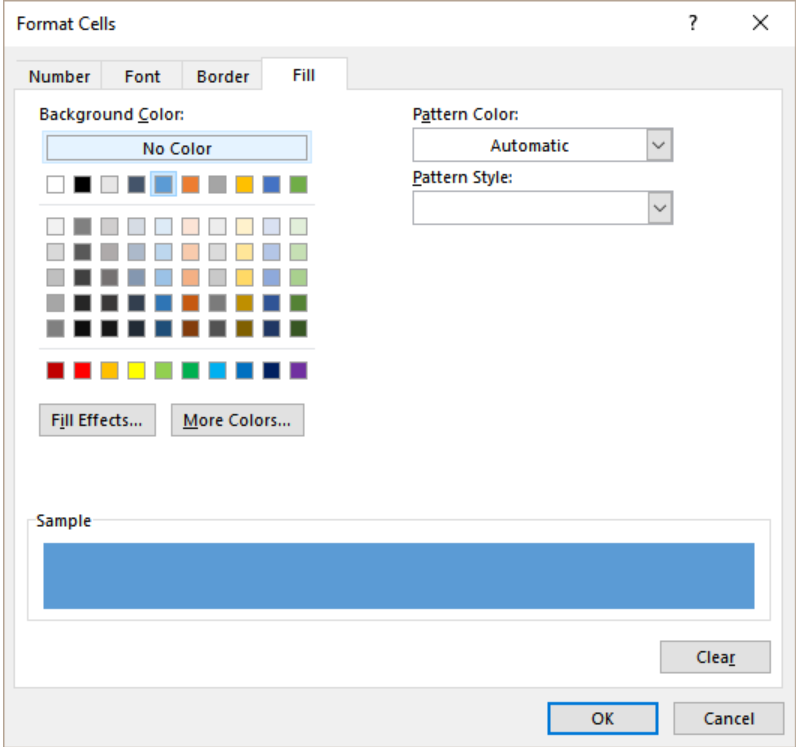Figure 5. Setting the Format of Display

• Click Ok. This will redirect us back to the previous formula box. We need to click Ok for the last time.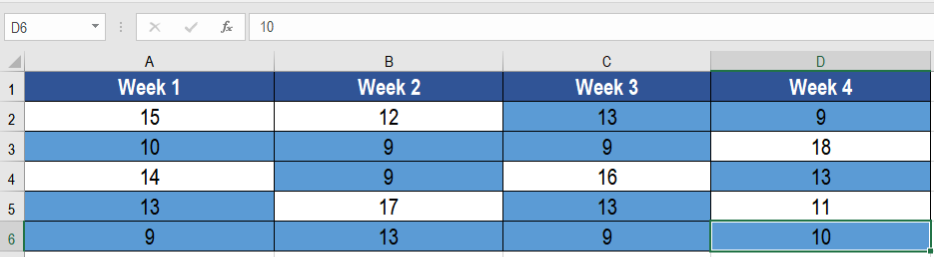Figure 6. Example of the Final Result

This will highlight the duplicate cells containing the temperatures 9,10 and 13 in columns A to D.

Most of the time, the problem you will need to solve will be more complex than a simple application of a formula or function. If you want to save hours of research and frustration, try our live Excelchat service! Our Excel Experts are available 24/7 to answer any Excel question you may have. We guarantee a connection within 30 seconds and a customized solution within 20 minutes.

### Did this post not answer your question? Get a solution from connecting with the expert.Another blog reader asked this question today on Excelchat:
Solution examplesI need a formula to count the number of rows that contain at least one of two possible phrases, but not give me the total number of instances that both phrases occur overall.
Solved by E. B. in 33 minsI want to be able to alter the appearance (font color and fill) of a column of numbers using conditional formatting, based on being greater than or less than the numbers from another column. I am using Excel 2016. I am unable to get any formula to work. Please help!
Solved by I. J. in 59 minsI need assistance with an excel formula. I have a 12 month sheet that has a summary page on the 13 page. the monthly totals changes so on the summary page I want to sum the difference - eg. if Jan total is 1500, feb total is 1000, mar total is 800, I want the summary page to show the current amount of 800 when you place the formula to capture all months---showing the diminishing value rather than the sum of all the pages
Solved by E. L. in 60 minsi have a table with Dates, First names , Last names and i want to count how many times a name occurs, but if a name occurs more than once on a particular date i need to only count it once. this is on excel and not google sheets
Solved by F. H. in 31 minsNeed a formula that counts (1,2,3,4 ect..in cell M21) a range of cells, N4:N20, which are annual premium values. But I only want to add a sale count if the average monthly premium is above \$30 per sale. So if someone has 4 sales but they don't equal a total of \$120/mth (\$30 x 4 = \$120)... they would only get a count of 3 sales.
Solved by S. E. in 60 mins## Subscribe to Excelchat.coAnother blog reader asked this question today on Excelchat: